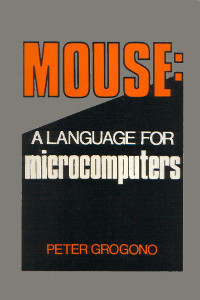## Thursday, 23 August 2012

### Mouse: a Language for Microcomputers by Peter GrogonoMouse is a stack-based interpreted programming language descended from MUSYS, an earlier language for a DEC PDP/8 with 4096 words of memory.

In Mouse: a Language for Microcomputers, Grogono defines the language and develops an intepreter step-by-step as he introduces each feature of the language.

The first few chapters cover the basics: postfix expressions, variables, control structures, pointers and macros. Later chapters include two complete Mouse interpreters, one in Pascal, the other in Z80 assembly language.

Here's a brief summary of the Mouse language. Instructions pop their operands from and push their result on the stack. ( before -- after ) shows the stack effects of each operation.

### Maths / Logic

Mouse is looking pretty Forthlike so far! `\` is the equivalent of `MOD` in Forth:

• `+` - ( x y -- z ) calculate z, the sum of x+y
• `-` - ( x y -- z ) calculate z, the difference of x-y
• `*` - ( x y -- z ) calculate z, the product of x×y
• `/` - ( x y -- z ) calculate z, the quotient of x/y
• `\` - ( x y -- z ) calculate z, the remainder of x/y
• `<` - ( x y -- z ) if x<y then z is true, otherwise false
• `=` - ( x y -- z ) if x=y then z is true, otherwise false
• `>` - ( x y -- z ) if x>y then z is true, otherwise false

### Input / Output

Only `?` doesn't have an exact equivalent in Forth. `?'` is the same as Forth's `KEY`. `!'` is the same as `EMIT` and `!` is `.`:

• `?` - ( -- x ) read a number x from the keyboard
• `?'` - ( -- x ) read a character x from the keyboard
• `!` - ( x -- ) display a number x
• `!'` - ( x -- ) display a character x
• `"…"` - ( -- ) display the quoted string

### Peek and Poke

`:` is the equivalent of `!` in Forth. `.` is the equivalent of `@`:

• `:` - ( x addr -- ) store x in address addr
• `.` - ( addr -- x ) read x from address addr

### Control Structures

`[ … | … ]` is similar to Forth's `IF … ELSE … THEN`.

`( … ↑ … )` is similar to Forth's `BEGIN … WHILE … REPEAT`:

• `[` - ( x -- ) if x is false, jump to the matching `|` or `]`
• `|` - ( -- ) jump to the matching `]` (not always implemented)
• `]` - ( -- ) end a `[ … | … ]` structure
• `(` - ( -- ) start a loop
• `↑` - ( x -- ) exit loop if x is false (often rendered as `^`)
• `)` - ( -- ) end loop, jump back to matching `(`
• `~` - ( -- ) the remainder of the line is a comment

### Macro Definitions

• `#x;` - ( -- ) call macro x
• `\$x` - ( -- ) define macro x
• `@` - ( -- ) end macro definition
• `%` - ( x -- z ) access macro parameter

Macros are the Mouse equivalent of subroutines. A macro is defined by `\$x … @` and called with `#x;`. Parameters can be passed between the macro name and semicolon. For example `#x,7,5,9;` will pass the parameters 7, 5 and 9 to x.

A macro accesses it's parameters using `%`: `1%` for the first, `2%` for the second, etc. A parameter is evaluated every time it's accessed and can be almost any valid Mouse code.

A macro has 26 local variables, A to Z. 26 macro names are available, A to Z.

### Example Code

Here are a few classic examples:

• Hello World (which recently celebrated it's 40th birthday)
• Fibonacci Numbers (the typical bad example of recursion)
• Greatest Common Divisor (a better example of recursion)

#### Hello, World

```"Hello, World!"\$ ```

Displays the string Hello, World. The exclamation mark isn't printed. An exclamation in a string instructs the interpreter to print a line break. All Mouse programs end with `\$`.

#### Fibonacci Numbers

```\$F 1% N: ~ store parameter in N N. 2 < [ N. ] ~ if N < 2 then return N N. 1 > [ #F, N. 1 - ; ~ otherwise calculate F(N-1) #F, N. 2 - ; ~ | and F(N-2) + ] ~ | and return their sum @ ```

`F` calculates Fibonacci numbers using the recurrence relation Fn = Fn-1 + Fn-2 with F0 = 0, F1 =1. Note this is really slow, Ο(φn). Calculating F40 takes 6 minutes. There are better ways to calculate Fibonacci numbers!

#### Greatest Common Divisor

```\$G 1% X: 2% Y: ~ store parameters X. Y. = [ X. ] ~ if X = Y then GCD = X X. Y. > [ #G, X. Y. - , Y. ; ] ~ otherwise subtract the X. Y. < [ #G, Y. X. - , X. ; ] ~ | smallest from the @ ~ | largest and recurse ```

Another example of recursion. `G` calculates the GCD using Dijkstra's method.